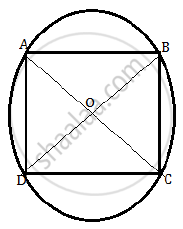# All the vertices of a rhombus lie on a circle. Find the area of the rhombus, if the area of the circle is 1256 cm^2 - Mathematics

All the vertices of a rhombus lie on a circle. Find the area of the rhombus, if the area of the circle is 1256 cm2. [Use p = 3.14]

#### Solution

Given that the area of the circle is 1256 cm2.

pir^2=12563.14xxr^2

3.14xxr^2=1256

r^2=1256/3.14

r^2=400

r=20 cm

If all the vertices of a rhombus lie on a circle, then the rhombus is square.
Consider the following figure.Here A, B, C and D are four points on the circle.
Thus, OA = OB = OC = OD = radius of the circle.
⇒AC and BD are the diameters of the circle.
By Pythagoras theorem, we have,

2AD^2=(2x20)^2....[AD=CD

2AD^2=(40)^2

AD^2=1600/2

AD^2=800 cm^2

If AD is the side of the square, then AD2 is the area of the square.

Thus area of the square is 800cm2

Concept: Circumference of a Circle
Is there an error in this question or solution?